gbPoints
gbPoints provides an incredibly simple way to plot 3D points. Just paste in a list of points, given by XYZ coordinates and gbPoints will plot the data. You can adjust or view the resulting plot in a variety of ways, including manual and automatic rotation of the image.

gbPoints can plot mesh lines between arrays of points (in Version 2, gbPoints will recognize Lines and Triangles model data).

Key gbPoints features include:

 Plots 3D data points (Ver 2 will include line and triangle model data) Built-in equation editor to generate points of form Z=f(x,y) Manual and automatic rotation of models on any axis Manual creation of models (type in point/line/triangle data) Mouse-based creation of models Lathe mode for automatic generation of symmetric models Online Help Online Update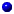Download (ver 1.1, 0.8M) (change history)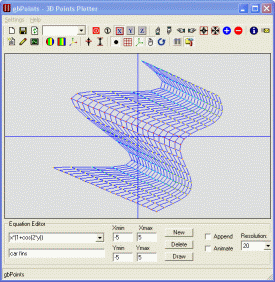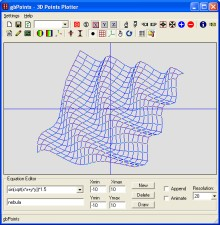gbPoints consists of a single window with four main areas - toolbars, model data listing, 3D image picturebox, and Equation Editor.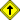Return to top of document

### Toolbar OptionsThe following functions are available on the top toolbar:
• New
creates an empty model
• Save As
saves the current model to a file
• Refresh
restores model to content of Model Data Listing
• Models
dropdown of available model files
• Allow Rotation
toggles automatic rotation
• Single Step Rotation
rotates model through a single angle of rotation
• X, Y, Z
Toggles automatic rotation about the respective axis
• Up / Down / Left / Right
moves the model the indicated direction
• Center
centers the model about the coordinate axes
• Fit to Size
sizes model to fit within the current window
• Zoom In
expands the model to fill more of the screen
• Zoom Out
reduces the model to fill less of the screen
• Information
displays model information in a popup window
• Email Author
allows user to send email to gbPoints author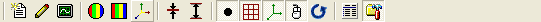The following functions are available on the bottom toolbar:

• Lathe Mode - New Model
clears the model and puts gbPoints into Lathe mode
• Lathe Mode - Edit Existing Points
puts gbPoints into Lathe mode
• Lathe Mode - Apply Lathe
applies lathe to all existing points
• Points Color
sets default point color
• Background Color
sets background color of image
• Axis Color
sets axis color
• Decrease Z
Decreases the Z-values of the model
• Increase Z
Increases the Z-values of the model
• Display Points
Displays the points of the model
• Display Mesh
Displays mesh (lines between points)
• Display Axes
Display the X-Y axes
• Allow Mouse Rotation
Allows the user to rotate the model using a mouse
• Reverse Rotation Direction
Reverse the direction of rotation
• Display Model Data
Displays a listing of all model data on the left side of the window
• Display Equation Editor
Displays the Equation Editor at the bottom of the window

Model Data Listing
gbPoints models are stored in a text file. The first two lines consist of the title (line 1) and model setting (line 2), as follows:

• Title
simple single line of text
• Settings
five properties, separated by spaces, as follows:
• # Points
• # Lines
• # Triangles
• MeshX (mesh width in number of points)
• MeshY (mesh height in number of points)

The rest of the text file consists of any number of the following lines, corresponding to data about points, lines and triangles:

• Pxxx X-position Y-position Z-position color
• Lxxx point1 point2 color
• Txxx point1 point2 point3 color

An sample of lines from the galaxy.txt sample model are:

```    Galaxy
500 0 0 22 22
P0 -1793 -2 2818 255
P1 2355 0 -1732 255
P2 1012 17 -1732 255
P3 -184 -603 195 255
P4 919 -910 -106 255
P5 2377 -5 864 255
P6 -129 0 6191 255
```

Note: gbPoints reads, and numbers, points/lines/triangles as it reads them in the model text file. The number behind the "P/L/T" is not necessary and will be ignored by gbPoints. Using it does provide the user with an easier way to manually edit or check data in the model file.

Equation Editor
gbPoints is distributed with a sample set of equations. The equations can be accessed using the dropdown list. The list of equations is found in the file 'gbEquations.txt', located in the gbPoints installation directory. The file can be edited manually. Or, equations may be edited using the Equation Editor.Any existing equation can be edited by simply changing the equation in the textbox of the dropdown list. Changes made to an equations are saved automatically when the equation is Drawn.

The Equation Editor contains the following settings:

• Equation
equation text string (see below for allowable content)
• Title
text title of the equation
• Xmin/Xmax/Ymin/Ymax
range over which the equation will be plotted
• New
adds a new equation (x+y is used as the default equation)
• Delete
deletes the current equation
• Draw
plots the equation according to the current settings
• Append
adds the equation to the current model (plots on top of the existing model)
• Animate
repeatedly plots the equation with varying settings
• Resolution
determines how many divisions are used to plot the equation

The equation editor supports the following Operators and General Functions:

Operators:

```+     ^     !     <=
-     %     >     !=
*     &     >=    ==
/     |     <
```

General functions:

```abs            cosh       max(x,y,z,..)    sum(x,y,z,..)
acos           fact       min(x,y,z,..)    tan
asin           floor      rand()           tanh
atan           hex        rand(min,max)
avg(x,y,z,..)  if         round
bin            isNaN      sin
ceil           log        sinh
cos            log10      sqrt
```